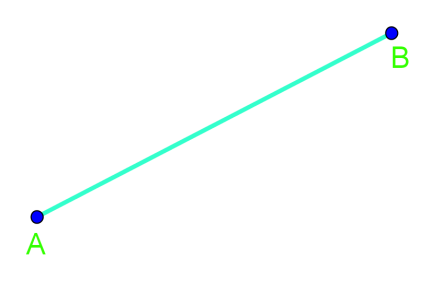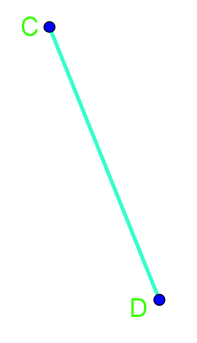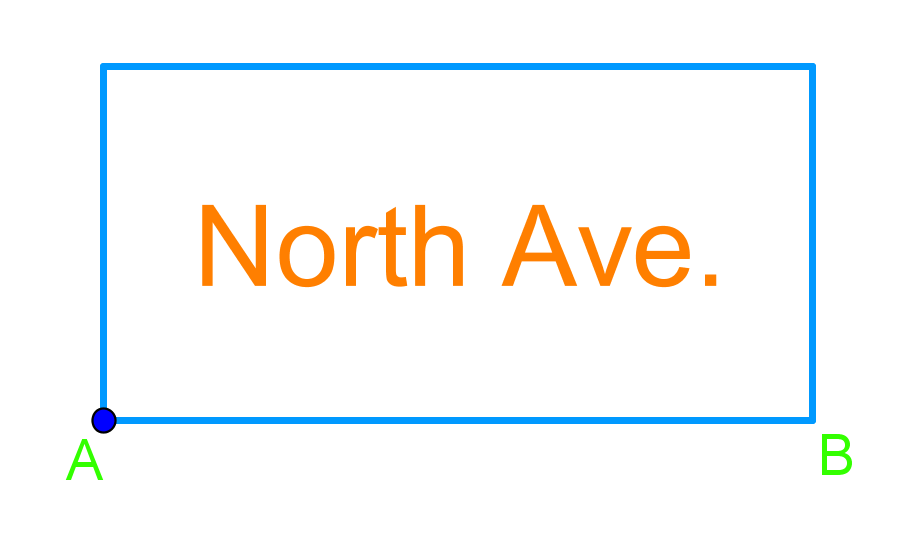# Perpendicular bisectors#### All in One Place

Everything you need for better grades in university, high school and elementary.#### Learn with Ease

Made in Canada with help for all provincial curriculums, so you can study in confidence.#### Instant and Unlimited Help

0/2
##### Intros
###### Lessons
1. How to use a protractor?
• What is a perpendicular bisector?
• How to draw it?
0/3
##### Examples
###### Lessons
1. Draw the perpendicular bisector of the line below.

1.2.2. Line AB below represents the bottom edge of a sign. The sign needs a perpendicular bisector support so that it can be put up on the corner of North Avenue. Draw the support.###### Topic Notes
In previous section, we were introduced to parallel and perpendicular line segments. In this section, we will expand our knowledge on this topic by learning how to draw perpendicular bisectors. Perpendicular bisectors are found everywhere in the world around us. For example, engineers use perpendicular bisectors when designing support beams for structures, including buildings and bridges.

## What is a bisector

### bisect definition

The definition of bisector is something that cuts an object into two equal parts. This "something" is usually a line or a segment.

## What is a perpendicular bisector

The perpendicular bisector definition tells us that it helps us to find the midpoint of a line.

### Perpendicular bisector theorem

When we have a perpendicular bisector, we can put the perpendicular bisector theorem into use. The perpendicular bisector theorem tells us that if a point is on the perpendicular bisector of a segment, it is then equidistant from the endpoints of that segment.

The reverse is also true in that if a point is equidistant from a segment's endpoints, it then lies on the perpendicular bisector.

We learn from this theorem that there's a series of points that are equidistant from the endpoints of a line segment which collectively can form a line to the midpoint of this line segment at a right angle.

## How to find perpendicular bisectors

In order to find a perpendicular bisector, it's quite simple. You may have guessed how to do this after the above section that explains what a perpendicular bisector is.

A straightforward way of finding a perpendicular bisector is to measure a line segment that you need to bisect. Then divide the measured length by two in order to find its midpoint. Draw a line out from this midpoint at a 90 degrees angle. That's all you'll need to do to find the perpendicular bisector if you've got a ruler!

## Example problems

If you're ready to try finding a perpendicular bisector, let's put the above steps we learned to use through example problems.

Question 1:

Draw the perpendicular bisector of the line below

Solution:

The first step to finding a perpendicular bisector is measuring the line segment you're given.

In the video example, we've found the measurement of the line to equal 14 cm. So we construct the perpendicular bisector according to this length. This cuts the line into two equal 7 cm parts with a midpoint between them. From this midpoint, extend it outwards at a 90 degrees angle. The black line in the diagram belows shows you the perpendicular bisector that is your answer.

Question 2:

Line AB below represents the bottom edge of a sign. The sign needs a perpendicular bisector support so that it can be put up on the corner of North Avenue. Draw the support.

Solution:

Once again, begin by measuring the line AB. Find the center between A and B to get the midpoint—the halfway point on the line. Lastly, draw a line out from that midpoint at a 90 degree angle.

In the end, we'll get this as your final answer after you've bisected AB:

To look more into perpendicular bisectors, see this interactive figure that helps you see how a perpendicular bisector is constructed when you've got a line with two endpoints. If you're still not sure about these bisectors, try revising your introduction to parallel and perpendicular line segments.

If you wanted to further your learning, feel free to check out the lessons on parallel line equations and perpendicular line equations that puts what you've learned here into use.